# Turning images into ridgeline plots

What if we turn images into ridgeline plots?

# Ridgeline plots

“Ridgeline plots are partially overlapping line plots that create the impression of a mountain range. They can be quite useful for visualizing changes in distributions over time or space.” - Claus Wilke.

They have been quite popular recently. Some references include:

• The work of James Cheshire to represent population density
• Alex Whan’s post, reproducing the Joy Division’s album cover
• The ggridges package from Claus Wilke
• In Python, the recent ridge_map library by Colin Carroll finally convinced me to perform some experiments

# Import images

First, let’s load the required packages. Then, we create a function to import an image from a url and store the grayscale pixel values as a matrix.

``````library(data.table)
library(ggplot2)
library(ggridges)
library(jpeg)

img_to_matrix <- function(imgurl) {
tmp <- tempfile()
file.remove(tmp)
img <- t(apply(img, 2, rev)) # rotate
print(paste("Image loaded.", nrow(img), "x", ncol(img), "pixels."))
img
}``````

We’ll use two images throughout this post.

``````url1 <- "http://upload.wikimedia.org/wikipedia/en/8/86/Einstein_tongue.jpg"

img1 <- img_to_matrix(url1)``````
``##  "Image loaded. 230 x 286 pixels."``
``img2 <- img_to_matrix(url2)``
``##  "Image loaded. 391 x 480 pixels."``
``````par(mfrow = c(1, 2))
image(img1, col = gray.colors(50))
image(img2, col = gray.colors(50))``````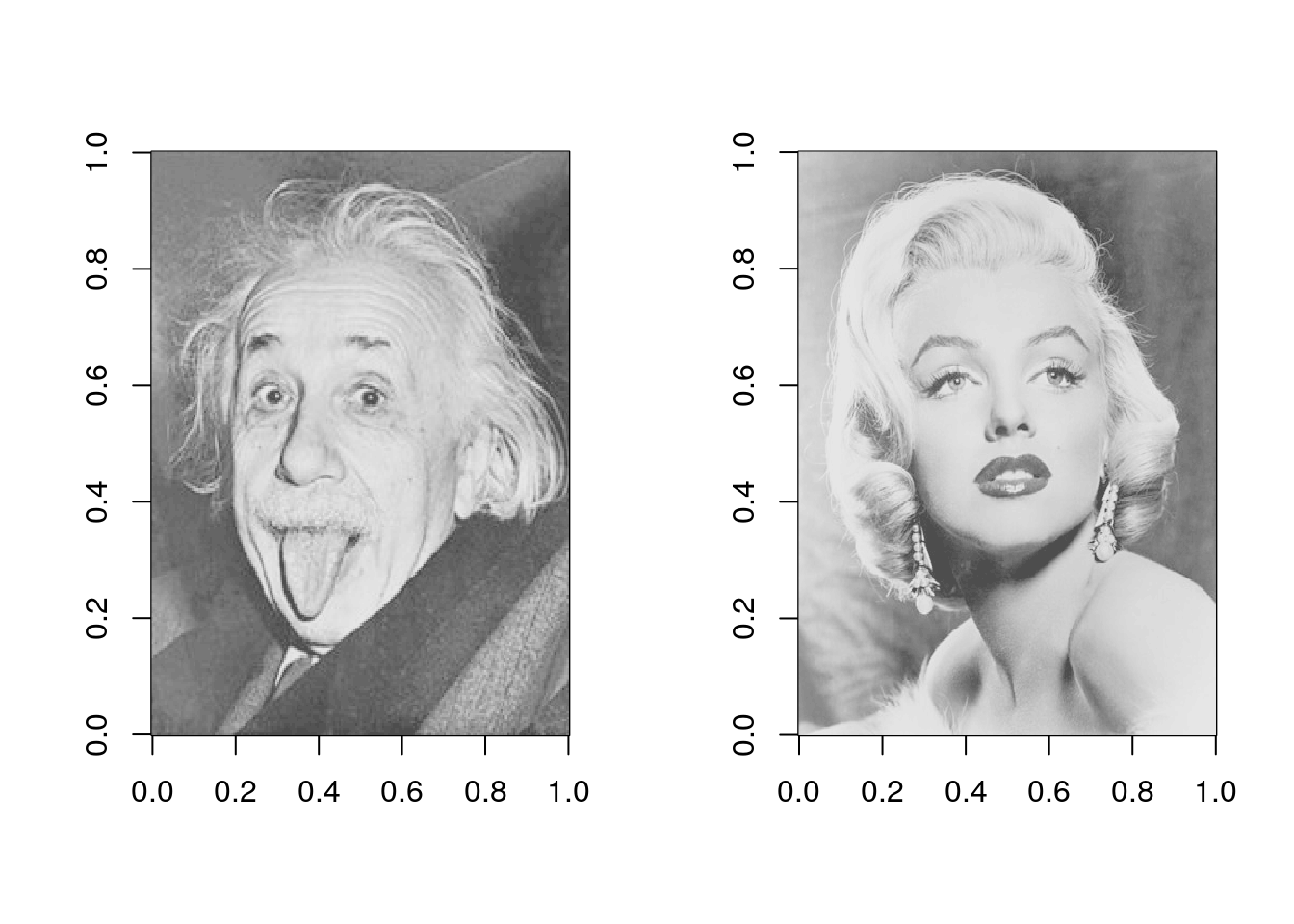## Convert image to a data.frame

Next, the `matrix_to_dt` function will convert an image matrix into a data.frame. The data.table package is used here for convenience.
This function is simply used to melt the matrix. The coordinates on the y axis that are used below (`y2`) correspond to the y value + the pixel intensity (`z`).

This function has four parameters:

• `img`: the matrix object
• `ratio`: integer value, used to reduce the size of the matrix
• `height`: numeric value, scaling applied to pixel value, higher will make peaks taller (and create overlap)
• `y_as_factor`: boolean, will make things easier with ggplot2 `geom_ribbon()`
``````matrix_to_dt <- function(img, ratio = NULL, height = 3, y_as_factor = FALSE) {
if (!is.null(ratio)) {
img <- img[seq(1, nrow(img), by = ratio),
seq(1, ncol(img), by = ratio)]
}

imgdt <- data.table(x = rep(1:nrow(img), ncol(img)),
y = rep(1:ncol(img), each = nrow(img)),
z = as.numeric(img))

imgdt[, y2 := y + z * height]

if (y_as_factor) {
imgdt[, y := factor(y, levels = max(y):1)]
setorder(imgdt, -y)
}

imgdt[]
}``````

## Basic plots

Starting with a simple ggplot2 plot, using `geom_path()`.

``````imgdt <- matrix_to_dt(img1, ratio = 2L)

ggplot(data = imgdt) +
geom_path(aes(x     = x,
y     = y2,
group = y),
size = 0.15) +
theme_void()``````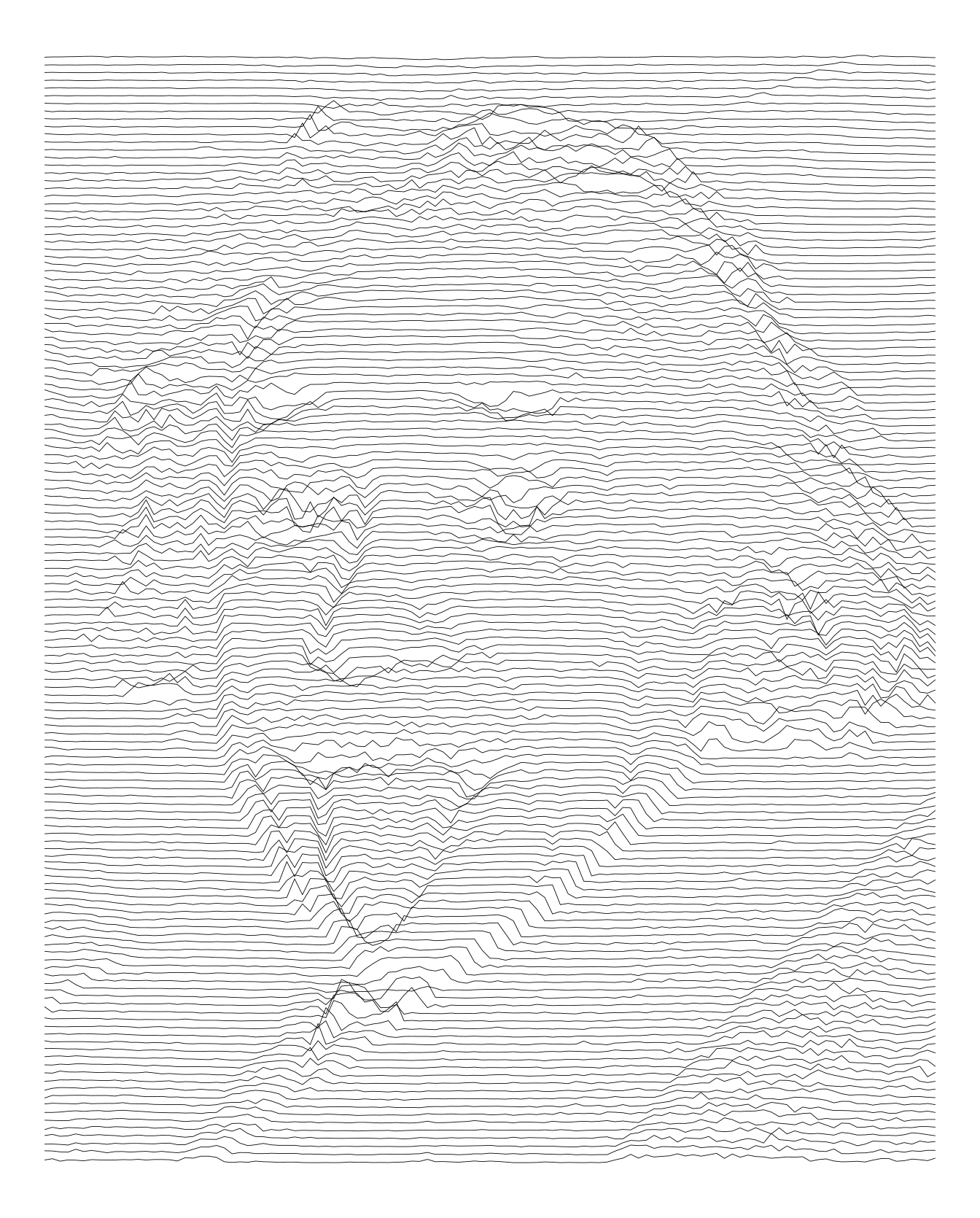Here is another try increasing the height.

``````imgdt <- matrix_to_dt(img1, height = 5L, ratio = 2L)

ggplot(data = imgdt) +
geom_path(aes(x     = x,
y     = y2,
group = y),
size = 0.2) +
theme_void()````````````imgdt <- matrix_to_dt(img2, height = 5L, ratio = 4L)

ggplot(data = imgdt) +
geom_path(aes(x     = x,
y     = y2,
group = y),
size = 0.2) +
theme_void()``````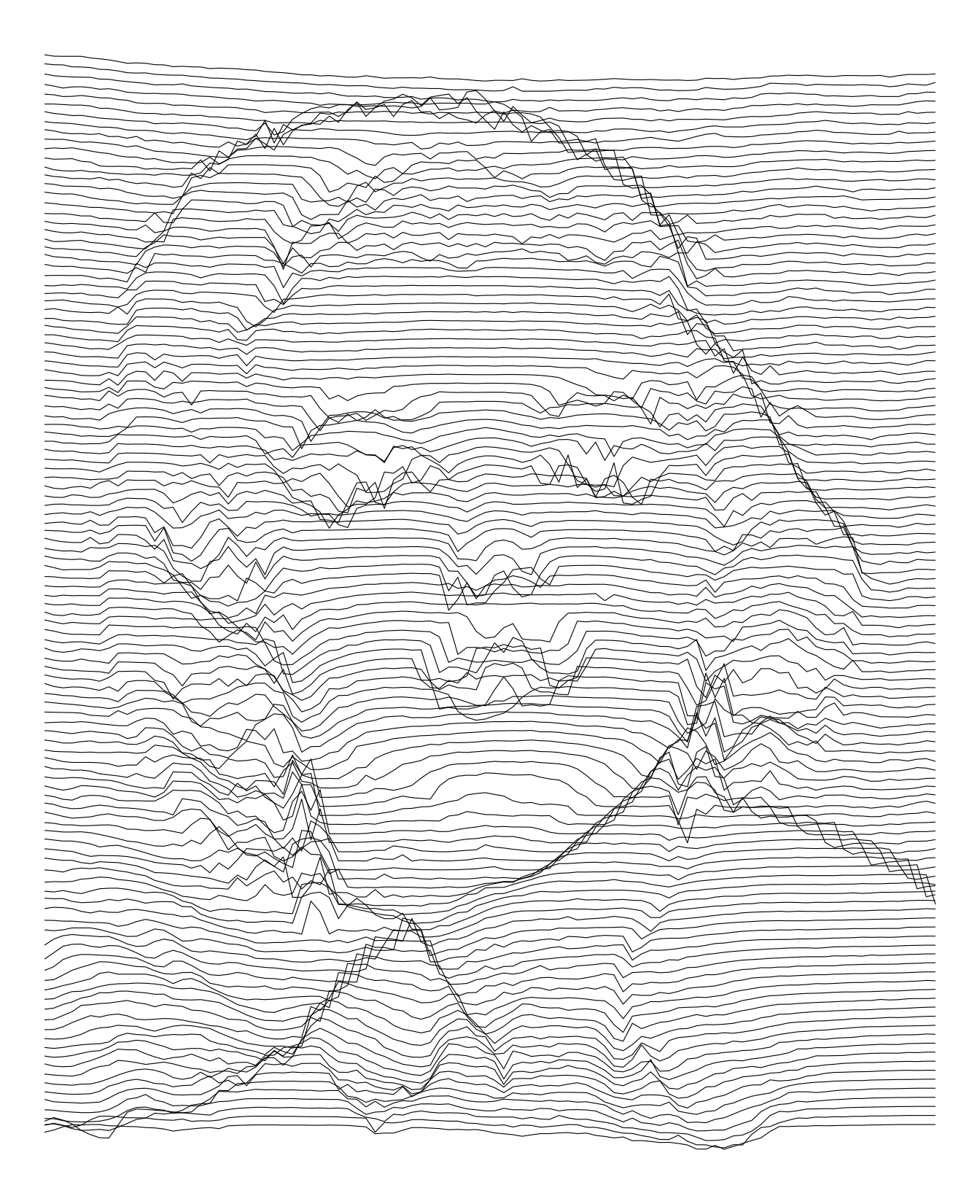We can also used vertical lines. In fact, I think they are more appropriate for these pictures.

``````imgdt <- matrix_to_dt(img1, height = 5L, ratio = 2L)

ggplot(data = imgdt) +
geom_path(aes(x     = x + z * 5L,
y     = y,
group = x),
size   = 0.15) +
theme_void()``````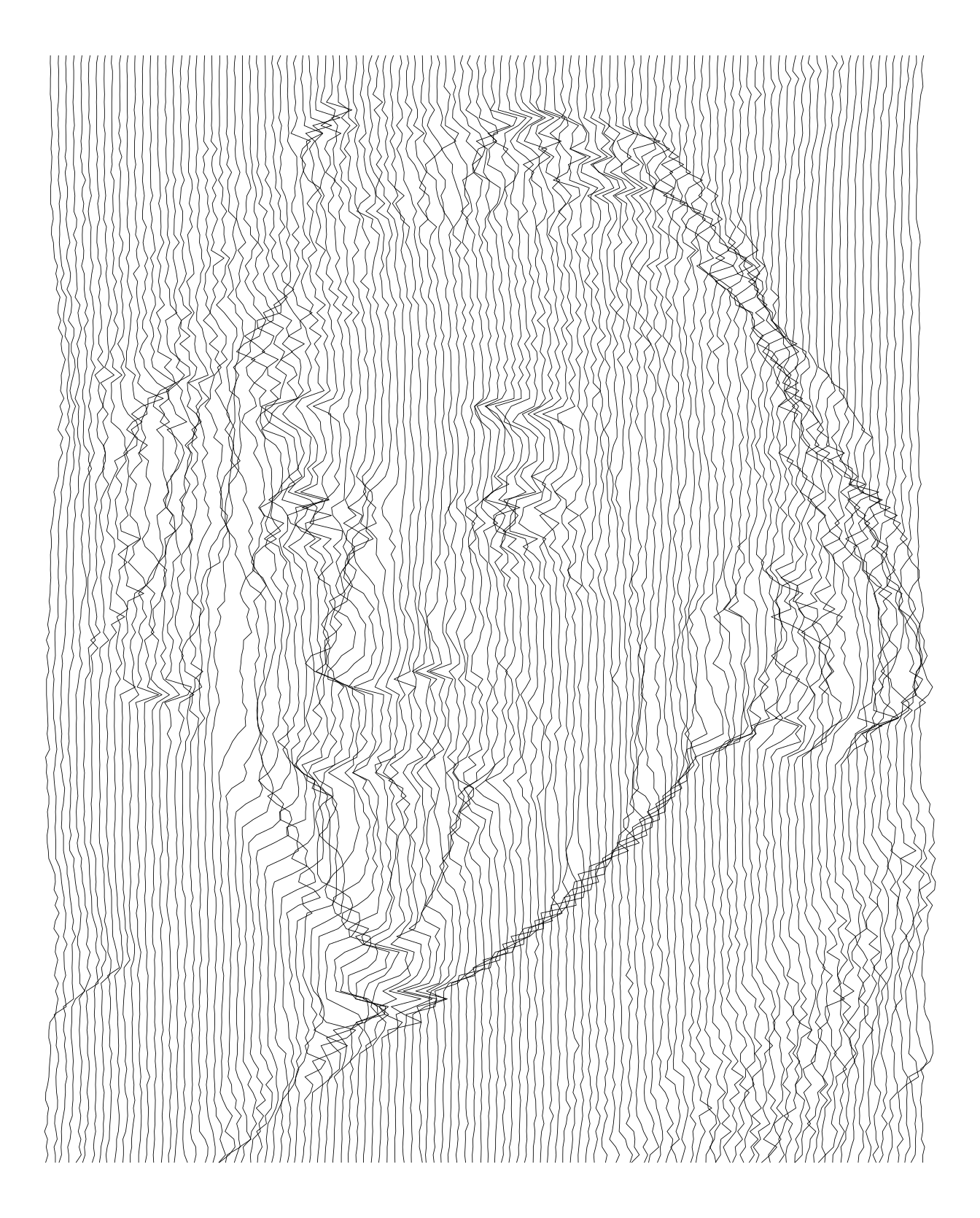``````imgdt <- matrix_to_dt(img2, height = 5L, ratio = 3L)

ggplot(data = imgdt) +
geom_path(aes(x      = x + z * 4L,
y      = y,
group  = x,
colour = x),
size = 0.2) +
scale_colour_continuous(type = "viridis", guide = FALSE) +
theme_void()``````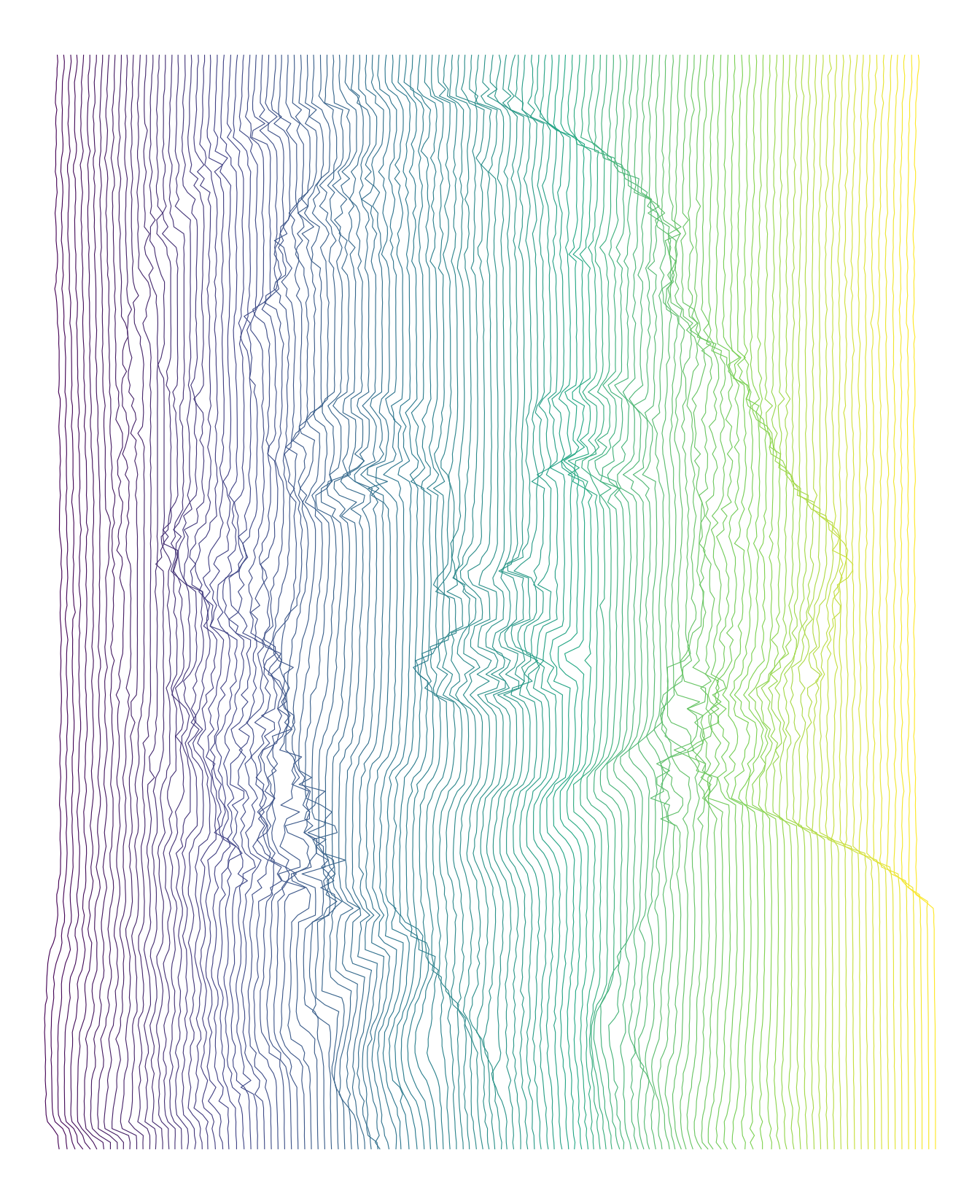## Ribbon plots

To prevent the lines from overlapping, we can use `geom_ribbon`. The y values are converted into factors to keep them in the right order.

``````imgdt <- matrix_to_dt(img1, height = 5L, y_as_factor = TRUE, ratio = 2L)

ggplot(data = imgdt) +
geom_ribbon(aes(x     = x,
ymax  = y2,
ymin  = 0,
group = y),
size   = 0.15,
colour = "white",
fill   = "black") +
theme_void()````````````imgdt <- matrix_to_dt(img2, height = 7L, y_as_factor = TRUE, ratio = 4L)

ggplot(data = imgdt) +
geom_ribbon(aes(x     = x,
ymax  = y2,
ymin  = 0,
group = y),
size   = 0.15,
colour = "white",
fill   = "black") +
theme_void()``````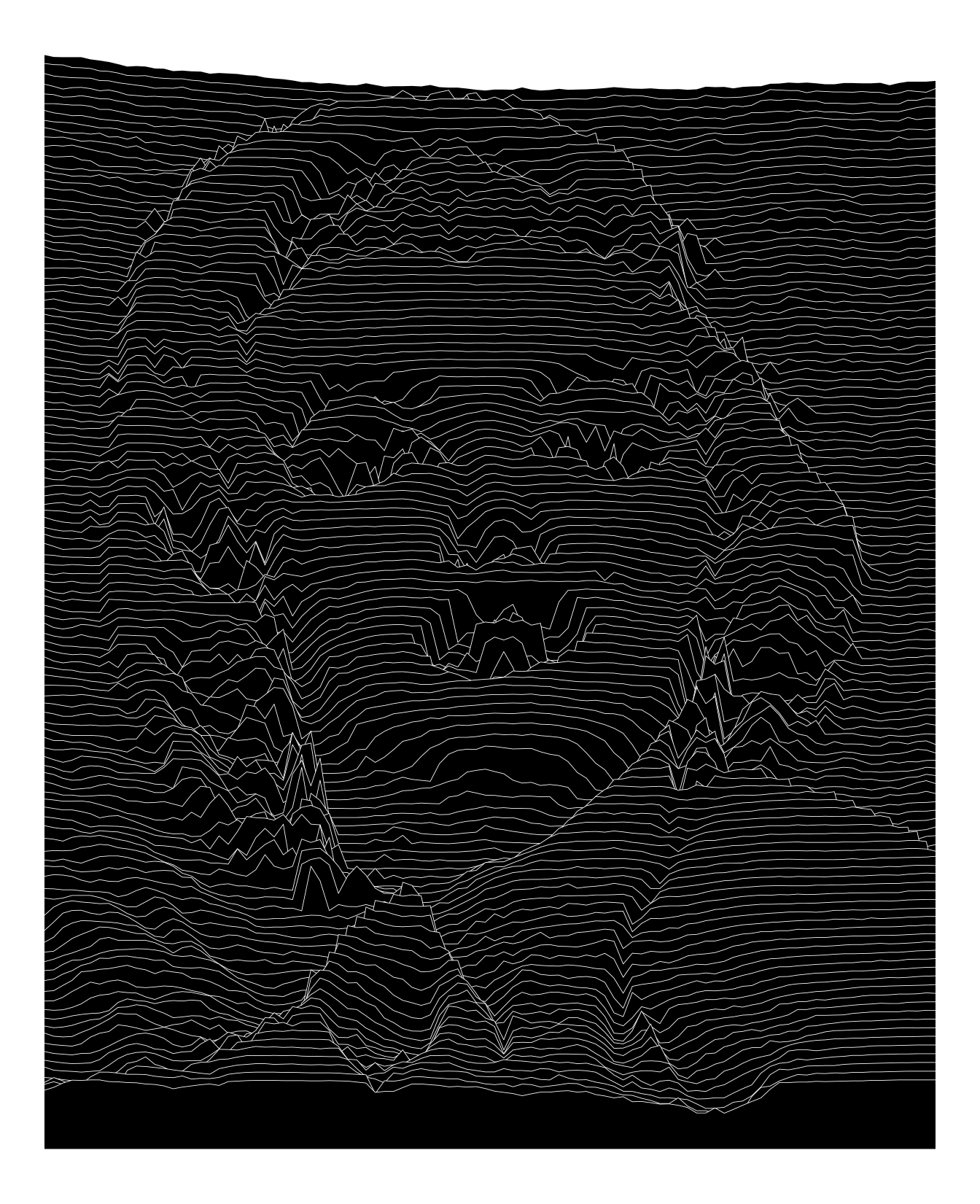## ggridges

The plots above are a bit sharp and lack the smooth aspect of ridgeline plots.
A first solution could be to use a smoothing spline. As an alternative, I gave a try to ggridges. The trick here is to transform the data by repeating the x values proportionally to the pixel intensity.

``````imgdt <- matrix_to_dt(img1, height = 7L, y_as_factor = TRUE, ratio = 2L)

imgdt2 <- imgdt[, .(x = rep(x, round(z * 100))), by = y]
imgdt2[, y := factor(y, levels = rev(levels(y)))]

ggplot(imgdt2,
aes(x = x,
y = y)) +
stat_density_ridges(geom      = "density_ridges",
bandwidth = 0.8,
colour    = "white",
fill      = "black",
scale     = 7,
size      = 0.15) +
theme_ridges() +
theme_void() +
theme(panel.background = element_rect(fill = 'black')) ``````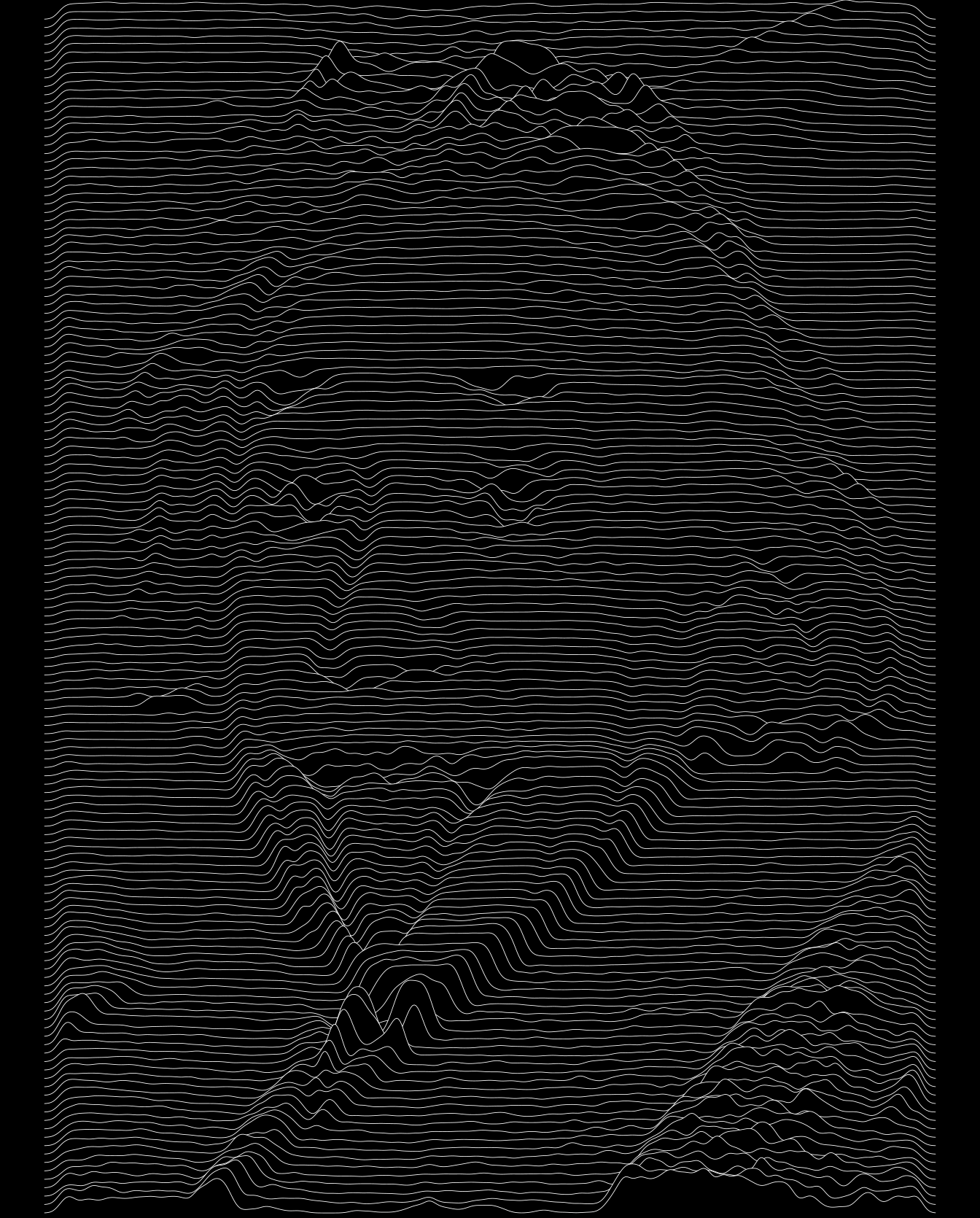``````imgdt <- matrix_to_dt(img2, height = 7L, y_as_factor = TRUE, ratio = 4L)

imgdt2 <- imgdt[, .(x = rep(x, round(z * 100))), by = y]
imgdt2[, y := factor(y, levels = rev(levels(y)))]

ggplot(imgdt2,
aes(x = x,
y = y)) +
stat_density_ridges(geom      = "density_ridges",
bandwidth = 0.8,
colour    = "white",
fill      = "black",
scale     = 5,
size      = 0.2,
rel_min_height = 0.15) +
theme_ridges() +
theme_void() +
theme(panel.background = element_rect(fill = 'black'))``````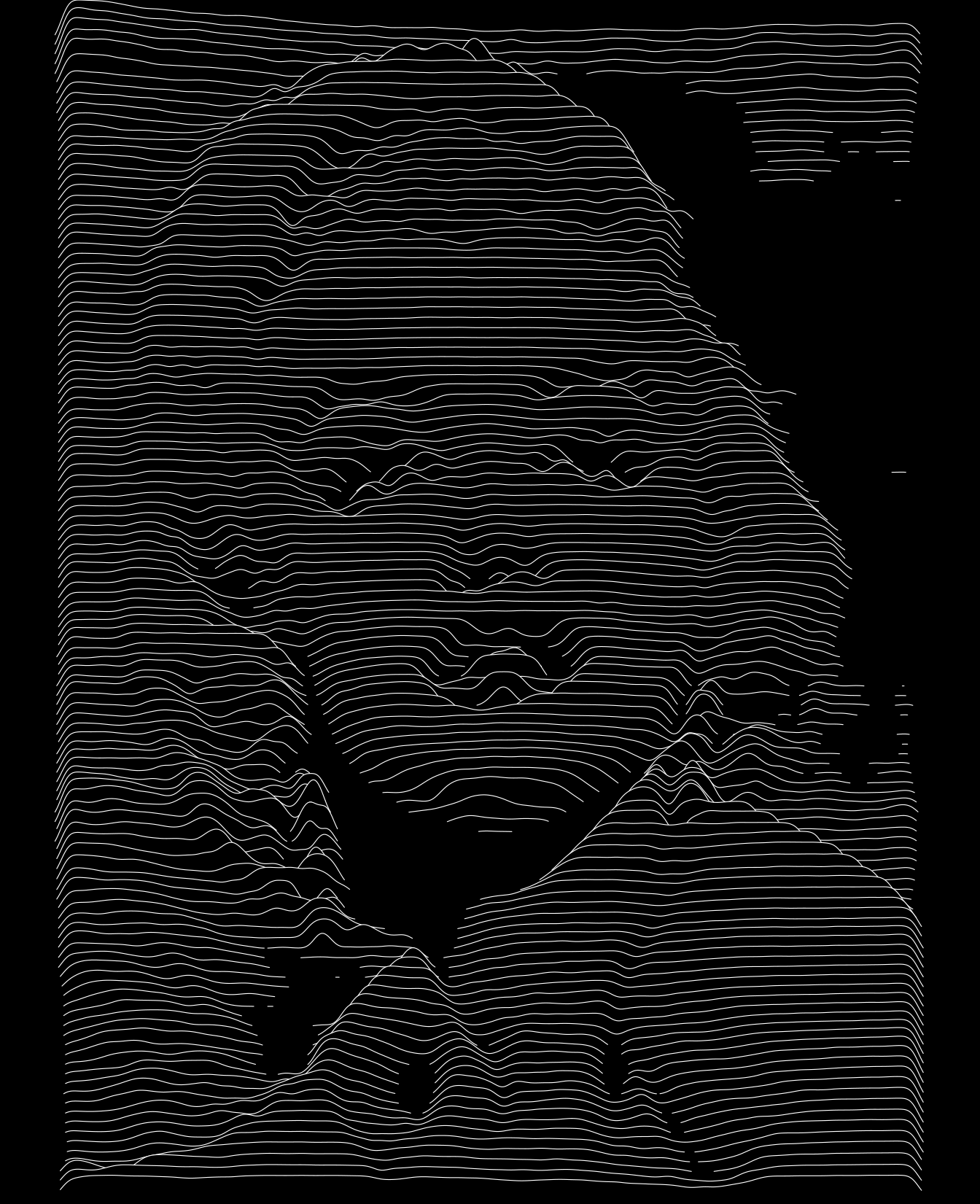``````ggplot(imgdt2,
aes(x = x,
y = y)) +
stat_density_ridges(geom      = "density_ridges",
bandwidth = 0.8,
alpha     = 1,
size      = 0.01,
fill      = "white",
color     = NA,
rel_min_height = 0.15) +
theme_ridges() +
theme_void() +
theme(panel.background = element_rect(fill = 'black'))``````## Conclusion

Zooming in and out the high-resolution plots rendered as a pdf file is mesmerizing and it is quite impressive to see the amount of details captured by these few intertwining lines.

Here is a final example.

``````jpeg("tmp.jpg")
plot(0, 0, type = "n", axes = FALSE, xlab = "", ylab = "")
text(0, 0, "ggplot2\nrocks!", cex = 9)
dev.off()``````
``````## png
##   2``````
``````img   <- readJPEG("tmp.jpg")
img   <- 1 - t(apply(img[, , 1], 2, rev))
imgdt <- matrix_to_dt(img, height = 4L, y_as_factor = TRUE, ratio = 3L)
file.remove("tmp.jpg")``````
``##  TRUE``
``````ggplot(imgdt) +
geom_ribbon(aes(x      = x,
ymax   = y2,
ymin   = 0,
group  = y,
colour = y),
size = 0.6,
fill = "white") +
theme_void() +
scale_colour_discrete(guide = FALSE)``````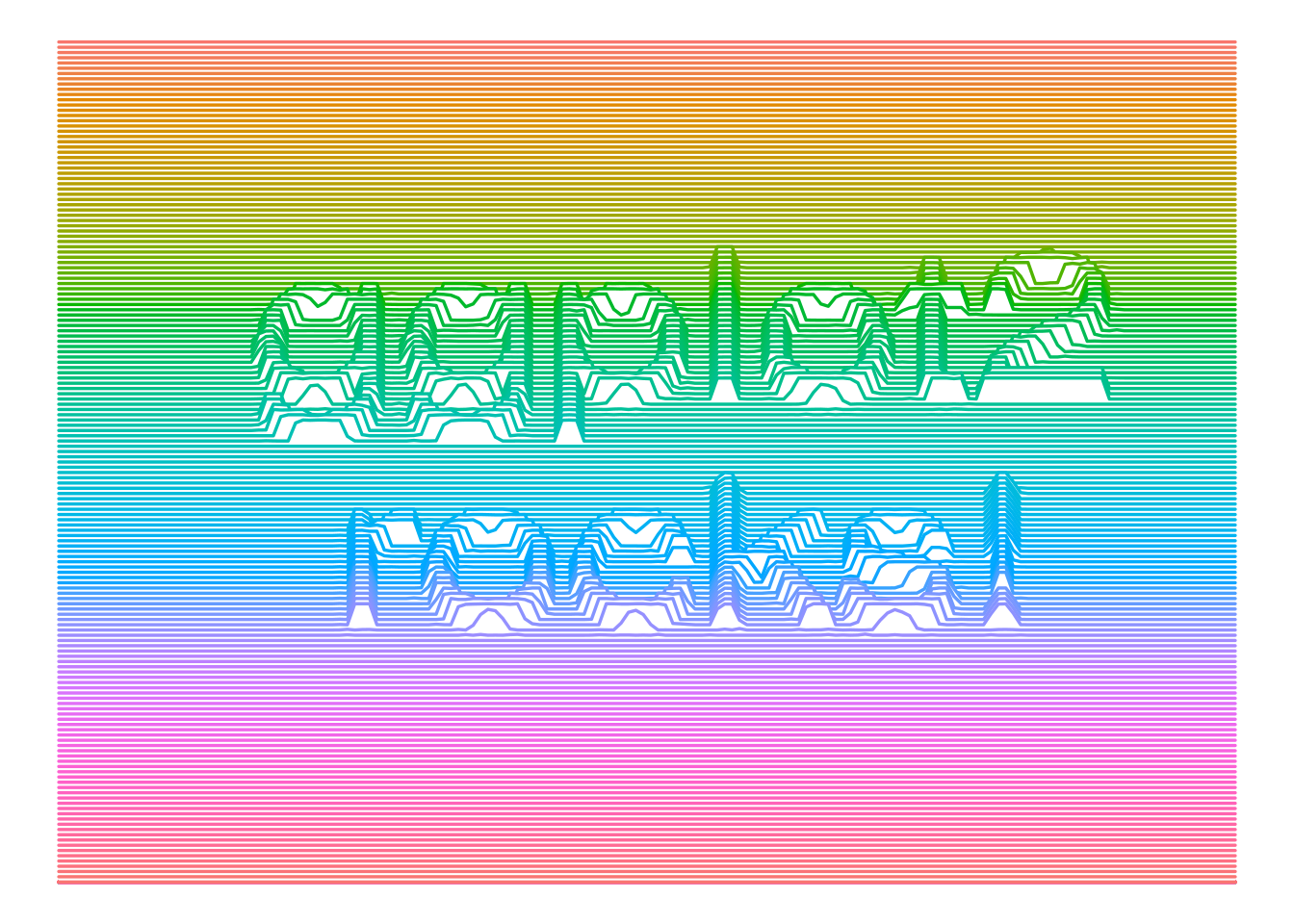``sessionInfo()``
``````## R version 3.6.3 (2020-02-29)
## Platform: x86_64-pc-linux-gnu (64-bit)
## Running under: Ubuntu 18.04.4 LTS
##
## Matrix products: default
## BLAS:   /usr/lib/x86_64-linux-gnu/blas/libblas.so.3.7.1
## LAPACK: /usr/lib/x86_64-linux-gnu/lapack/liblapack.so.3.7.1
##
## locale:
##   LC_CTYPE=en_US.UTF-8       LC_NUMERIC=C
##   LC_TIME=fr_FR.UTF-8        LC_COLLATE=en_US.UTF-8
##   LC_MONETARY=fr_FR.UTF-8    LC_MESSAGES=en_US.UTF-8
##   LC_PAPER=fr_FR.UTF-8       LC_NAME=C
##  LC_MEASUREMENT=fr_FR.UTF-8 LC_IDENTIFICATION=C
##
## attached base packages:
##  stats     graphics  grDevices utils     datasets  methods   base
##
## other attached packages:
##  jpeg_0.1-8.1      ggridges_0.5.2    ggplot2_3.3.1     data.table_1.13.0
##
## loaded via a namespace (and not attached):
##   Rcpp_1.0.3        pillar_1.4.3      compiler_3.6.3    plyr_1.8.5
##   tools_3.6.3       digest_0.6.23     viridisLite_0.3.0 evaluate_0.14
##   lifecycle_0.2.0   tibble_2.1.3      gtable_0.3.0      pkgconfig_2.0.3
##  rlang_0.4.6       yaml_2.2.0        blogdown_0.17     xfun_0.11
##  withr_2.1.2       stringr_1.4.0     dplyr_1.0.0       knitr_1.26
##  generics_0.0.2    vctrs_0.3.1       grid_3.6.3        tidyselect_1.1.0
##  glue_1.4.1        R6_2.4.1          rmarkdown_2.0     bookdown_0.16
##  purrr_0.3.3       farver_2.0.1      magrittr_1.5      scales_1.1.0
##  htmltools_0.4.0   colorspace_1.4-1  labeling_0.3      stringi_1.4.3
##  munsell_0.5.0     crayon_1.3.4``````